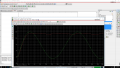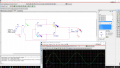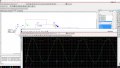# Need Help with Full wave rectifier in Pspice

#### MarsG

Joined Sep 12, 2015
24
My homework assignment is to design a Full Wave Bridge Rectifier (Attached the circuit I built in pspice and the full description of the assignment below.) The rectifier is to have a filter Capacitor across the Load.
Given:
Input = 120v-rms, 60Hz
Voltage Across individual diodes = .7V (1.4 together)

The Avg Outpt Voltage is to be 15v with a ripple of +- 1V peak

Calculations
120V-rms = 170v pk (Vrms= Vpk/sqrt2)

with the voltage drop from the diodes (1.4v)
The transformer has a ratio of 170:17 and a inductance ratio of (1/10)^2

The ripple frequency is double the source
60Hz----> 120Hz

The transient analysis does not seem right, can someone help me figure out the problem (wiring or maybe the wrong capacitor value).
I attached a screenshot below.

#### MikeML

Joined Oct 2, 2009
5,444
The filter capacitor must supply the total load current for ~8ms until the next current pulse arrives from the FWB.

To achieve a given amount of ripple, use the following method.

I*t = q = C*ΔV

Rearrange and solve for C

C=I*t/ΔV

ΔV (ripple) = 1V, so C= I*0.008/1 = 8*I mF = 8*(15/155)mF = 0.77mF = 770uF (Much bigger than you calculated...)

•Russmax

#### MarsG

Joined Sep 12, 2015
24
The filter capacitor must supply the total load current for ~8ms until the next current pulse arrives from the FWB.

To achieve a given amount of ripple, use the following method.

I*t = q = C*ΔV

Rearrange and solve for C

C=I*t/ΔV

ΔV (ripple) = 1V, so C= I*0.008/1 = 8*I mF = 8*(15/155)mF = 0.77mF = 770uF (Much bigger than you calculated...)
Thank you, Ihad originally put 800uF but thought it was wrong and took another approach, My marker was also in the wrong place.

#### shteii01

Joined Feb 19, 2010
4,644
The filter capacitor must supply the total load current for ~8ms until the next current pulse arrives from the FWB.

To achieve a given amount of ripple, use the following method.

I*t = q = C*ΔV

Rearrange and solve for C

C=I*t/ΔV

ΔV (ripple) = 1V, so C= I*0.008/1 = 8*I mF = 8*(15/155)mF = 0.77mF = 770uF (Much bigger than you calculated...)
I thought they said ripple is +-1 volt, would that make delta V = 2 volts?

#### MikeML

Joined Oct 2, 2009
5,444
I thought they said ripple is +-1 volt, would that make delta V = 2 volts?
The problem spec is somewhat ambiguous, but if so, that would cut the required cap in half...

#### Russmax

Joined Sep 3, 2015
82
I'm pretty sure the ripple spec means he cannot go below 14V. Or above 16V.
Regards

#### shteii01

Joined Feb 19, 2010
4,644
Oh, I misunderstood it, I thought it was +- 1 of 15V lol.
View attachment 91582
Your average is 15 volts. (14+16)/2=15 volts, that is your average. So the highest value you can have is 16 volts. The lowest value that allowed to have is 14 volts. The delta V is 2 volts, 1 volt above 15, 1 volt below 15. You must stay in this "band" between 14 and 16 volts.

#### MarsG

Joined Sep 12, 2015
24
Your average is 15 volts. (14+16)/2=15 volts, that is your average. So the highest value you can have is 16 volts. The lowest value that allowed to have is 14 volts. The delta V is 2 volts, 1 volt above 15, 1 volt below 15. You must stay in this "band" between 14 and 16 volts.
Should I use a lower input peak voltage at 160V to get a 15V at the output cause right now it seems im slightly above the 16V peak at output

#### shteii01

Joined Feb 19, 2010
4,644
Should I use a lower input peak voltage at 160V to get a 15V at the output cause right now it seems im slightly above the 16V peak at output
I think the ripple is given to you to figure out the smoothing capacitor. Just use MikeML formula to get the capacitor.

#### MarsG

Joined Sep 12, 2015
24
I think the ripple is given to you to figure out the smoothing capacitor. Just use MikeML formula to get the capacitor.
Is the sine wave supposed to drop under 0, cause the peak voltage from peak to peak is actually 17

#### shteii01

Joined Feb 19, 2010
4,644
Is the sine wave supposed to drop under 0, cause the peak voltage from peak to peak is actually 17
Forgive me for asking this, but: Why do you give a damn?
You are given an input.
You are asked to produce output.
You are given some details about what should be placed between input and output. Everything else is up to you. If the output of the transformer is something... Is that part of your assignment? Are you required to produce transformer output that have particular behavior? Because if you are... I can not find this part of your assignment. Are you holding out on us?

#### MarsG

Joined Sep 12, 2015
24
Forgive me for asking this, but: Why do you give a damn?
You are given an input.
You are asked to produce output.
You are given some details about what should be placed between input and output. Everything else is up to you. If the output of the transformer is something... Is that part of your assignment? Are you required to produce transformer output that have particular behavior? Because if you are... I can not find this part of your assignment. Are you holding out on us?
Not really, I just don't want to be marked off for anything so im including everything.

Is their any way to read the values on the simulation from the markers in order to get the current values.#### shteii01

Joined Feb 19, 2010
4,644
Not really, I just don't want to be marked off for anything so im including everything.

Is their any way to read the values on the simulation from the markers in order to get the current values.
View attachment 91587
Ok. About that sign wave. Check a few things:
1. Are you sure the signal has settled? I noticed in your assignment that they note that you want to wait for signal to enter steady state. So maybe if you wait, that signal will rise up and be, like you said, from 0 to 17 volts.
2. Check if the simulated components have some offset.
Is your input signal 120 VAC RMS centered on zero? Or is it from 0 to 120? Stuff like that.

You should be able to get current readings by placing current meter in series. So if you want current thought R1, put current meter in series with R1. If you want current through D2, put current meter in series with D2. And so on.

Finally. Remember that your diodes drop 0.7 volts each, 1.4 volts total. I think you noted that yourself. So you actually want output of the transformer to be 16+1.4=17.4 volts. I would be happier if I did not actually hit 16 or 14. Just stay inside the band, that is why they gave you that +-1 volt band to contain the ripple.

#### MarsG

Joined Sep 12, 2015
24
Ok. About that sign wave. Check a few things:
1. Are you sure the signal has settled? I noticed in your assignment that they note that you want to wait for signal to enter steady state. So maybe if you wait, that signal will rise up and be, like you said, from 0 to 17 volts.
2. Check if the simulated components have some offset.
Is your input signal 120 VAC RMS centered on zero? Or is it from 0 to 120? Stuff like that.

You should be able to get current readings by placing current meter in series. So if you want current thought R1, put current meter in series with R1. If you want current through D2, put current meter in series with D2. And so on.

Finally. Remember that your diodes drop 0.7 volts each, 1.4 volts total. I think you noted that yourself. So you actually want output of the transformer to be 16+1.4=17.4 volts. I would be happier if I did not actually hit 16 or 14. Just stay inside the band, that is why they gave you that +-1 volt band to contain the ripple.
How would I be able to center it at 0 in pspice? by using an offset?

#### shteii01

Joined Feb 19, 2010
4,644
How would I be able to center it at 0 in pspice? by using an offset?
Hm... I don't use PSpice. For general use I got old copy of MultiSim Textbook Edition that I got with a textbook back in 2000-2002.

#### MarsG

Joined Sep 12, 2015
24
Hm... I don't use PSpice. For general use I got old copy of MultiSim Textbook Edition that I got with a textbook back in 2000-2002.
Oh alright. since the output is supposed to be 15V at the load I just added the voltage drop from the diodes (1.4V) at the transformer secondary to be 16.4 and it came down closer to 15V. 169.7 : 16.4 ratio 120V-rms*sqrt(2)=169.7 peak#### shteii01

Joined Feb 19, 2010
4,644
Oh alright. since the output is supposed to be 15V at the load I just added the voltage drop from the diodes (1.4V) at the transformer secondary to be 16.4 and it came down closer to 15V. 169.7 : 16.4 ratio 120V-rms*sqrt(2)=169.7 peak
View attachment 91588
Yes, but now your load voltage drops below 14 volts and that is bad. Your ripple must be between 14 and 16 volts, that is requirement of your assignment.